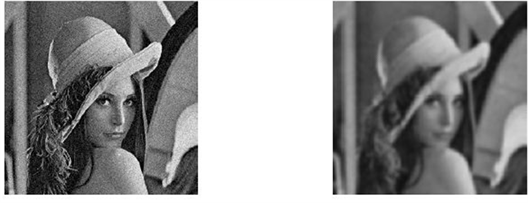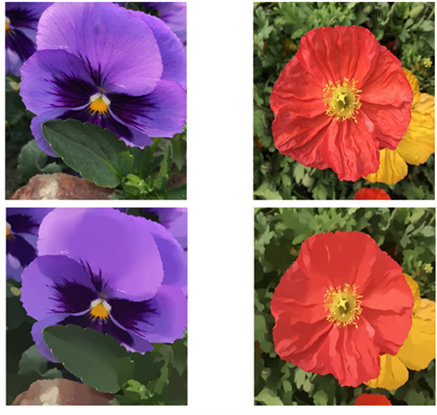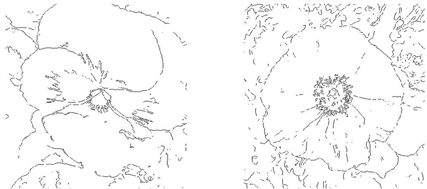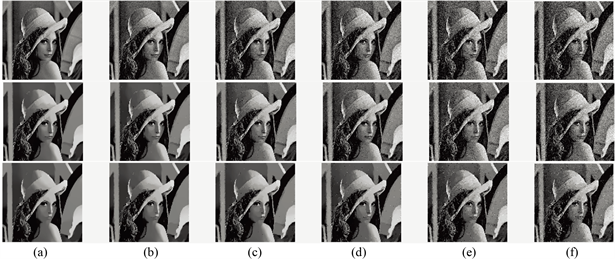三种常见的正则化图像处理模型研究Research on Three Common Regularized Image Processing Models

• 全文下载: PDF(836KB)    PP.622-631   DOI: 10.12677/AAM.2018.75074
• 下载量: 889  浏览量: 4,637   科研立项经费支持

Regularization is an important topic in inverse problems. Proper selection of regularization terms is essential for solving inverse problems. Image restoration is a typical inverse problem, so the discussion of regularization is also necessary for image restoration. Three regularization models, Tikhonov regularization, total variation regularization and gradient L0 regularization are intro-duced. Examples are given to restore grayscale and RGB images. It is found that denoising with Tikhonov regularization can produce blurring, while denoising with total variation regularization can preserve the boundary and the detail information; however, the L0 regularization smoothing is more sensitive to noise.

1. 引言

1.1 背景

1.2. 数字图像

1.3. 图像复原

$f=u+n$ (1)

$\underset{u}{\mathrm{min}}{‖u-f‖}_{2}^{2}$ (2)

2. 正则化图像复原方法

2.1. Tikhonov正则——用图像梯度的L2模的平方做正则化项

$\underset{u}{\mathrm{min}}\frac{1}{2}{‖\nabla u‖}_{2}^{2}+\frac{\lambda }{2}{‖u-f‖}_{2}^{2}$ (3)

$\lambda u-\Delta u=\lambda f$ (4)

$\Delta u\left(i,j\right)=u\left(i+1,j\right)+u\left(i-1,j\right)-4u\left(i,j\right)+u\left(i,j+1\right)+u\left(i,j-1\right)$ , $1 ,

$\Delta u\left(1,j\right)=u\left(2,j\right)-3u\left(1,j\right)+u\left(1,j+1\right)+u\left(1,j-1\right)$ , $1

$\Delta u\left(1,1\right)=u\left(2,1\right)-2u\left(1,1\right)+u\left(1,2\right)$ ,

$u\left(i,j\right)=\frac{1}{\lambda +4}\left(u\left(i+1,j\right)+u\left(i-1,j\right)+u\left(i,j+1\right)+u\left(i,j-1\right)\right)+\frac{\lambda }{\lambda +4}f\left(i,j\right)$

${u}^{0}=0$ ，利用Jacobi迭代法求解u，有：

${u}_{i,j}^{k+1}=\frac{1}{\lambda +4}\left({u}_{i+1,j}^{k}+{u}_{i-1,j}^{k}+{u}_{i,j+1}^{k}+{u}_{i,j-1}^{k}\right)+\frac{\lambda }{\lambda +4}{f}_{i,j},\text{\hspace{0.17em}}\text{\hspace{0.17em}}k=0,1,\cdots$ (5)

2.2. Total Variation (TV)正则——用图像的TV模(即图像梯度的L1模)做正则项

2.2.1. 背景

$\underset{u}{\mathrm{min}}{‖\nabla u‖}_{1}+\frac{\lambda }{2}{‖u-f‖}_{2}^{2}$ (6)

${u}_{k+1}=\underset{u\in BV\left(\Omega \right)}{\text{artmin}}\left\{{‖\nabla u‖}_{1}+\frac{1}{2\lambda }{‖g+{b}_{k}-u‖}_{2}^{2}\right\}$ (7)

2.2.2. 算法

2.3. 稀疏正则——用梯度的L0模做正则项

2.3.1. 背景

$\underset{S}{\mathrm{min}}{‖S-I‖}_{2}^{2}+\lambda C\left(S\right)$ (10)

$C\left(S\right)=$$\left\{p||{\partial }_{x}S{}_{p}|+|{\partial }_{y}{S}_{p}|\ne 0\right\}$ (11)

2.3.2. 算法

$\underset{S,h,v}{\mathrm{min}}\left\{\underset{p}{\sum }{\left({S}_{p}-{I}_{p}\right)}^{2}+\lambda C\left(h,v\right)+\beta \left({\left({\partial }_{x}{S}_{p}-{h}_{p}\right)}^{2}+{\left({\partial }_{y}{S}_{p}-{v}_{p}\right)}^{2}\right)\right\}$ (12)

$\underset{S}{\mathrm{min}}\left\{\underset{p}{\sum }{\left({S}_{p}-{I}_{p}\right)}^{2}+\beta \left({\left({\partial }_{x}{S}_{p}-{h}_{p}\right)}^{2}+{\left({\partial }_{y}{S}_{p}-{v}_{p}\right)}^{2}\right)\right\}$ (13)

$S={F}^{-1}\left(\frac{F\left(I\right)+\beta \left(F{\left({\partial }_{x}\right)}^{\ast }F\left(h\right)+F{\left({\partial }_{y}\right)}^{\ast }F\left(v\right)\right)}{F\left(1\right)+\beta \left(F{\left({\partial }_{x}\right)}^{\ast }F\left({\partial }_{x}\right)+F{\left({\partial }_{y}\right)}^{\ast }F\left({\partial }_{y}\right)\right)}\right)$ (14)

$\underset{h,v}{\mathrm{min}}\left\{\underset{p}{\sum }\left({\left({\partial }_{x}{S}_{p}-{h}_{p}\right)}^{2}+{\left({\partial }_{y}{S}_{p}-{v}_{p}\right)}^{2}\right)+\frac{\lambda }{\beta }C\left(h,v\right)\right\}$ (15)

$\underset{p}{\sum }\left\{\underset{{h}_{p},{v}_{p}}{\mathrm{min}}\left({\left({\partial }_{x}{S}_{p}-{h}_{p}\right)}^{2}+{\left({\partial }_{y}{S}_{p}-{v}_{p}\right)}^{2}\right)+\frac{\lambda }{\beta }H\left({h}_{p},{v}_{p}\right)\right\}$ (16)

$H\left({h}_{p},{v}_{p}\right)=\left\{\begin{array}{l}1\text{ }|{h}_{p}|+|{v}_{p}|\ne 0\\ 0\text{ }|{h}_{p}|+|{v}_{p}|=0\end{array}$ (17)

3. 数值算例左上图：256 × 256个像素的Lena图像；右上图：Tikhonov正则化去噪结果；左下图：TV正则化去噪结果；右下图：L0稀疏正则化结果

Figure 1. Noisy image and the processing results of a gray image左上图：512 × 512个像素的图像，添加了均方差sigma = 12的高斯白噪音；右上图：Tikhonov正则化结果；左下图：TV去噪结果；右下图：L0平滑结果

Figure 2. Noisy image and the processing results of a RGB images第一行图：545 × 500个像素的两幅图像；第二行图：L0平滑的结果；第三行图：对平滑后的图像提取的边界

Figure 3. L0 smoothing and sketching第一行：待平滑的图像，噪音程度见表1第一行；第二行：平滑参数取0.005时的平滑结果，PSNR值见表1第二行；第三行：平滑参数取0.01时的平滑结果，PSNR值见表1第三行

Figure 4. Images with different noise levels and their L0 smoothing resultsTable 1. Noise levels and PSNR values after L0 smoothing (unit: dB)

4. 结论和展望

1) 含有其他单个正则项的方法；

2) 含单个正则项的图像处理模型和含多个正则项的图像处理模型的对比分析。

  Rudin, L.I., Osher, S. and Fatemi, E. (1992) Nonlinear Total Variation Based Noise Removal Algorithms. Eleventh International Conference of the Center for Nonlinear Studies on Experimental Mathematics: Computational Issues in Nonlinear Science: Compu-tational Issues in Nonlinear Science, 60, 259-268. https://doi.org/10.1016/0167-2789(92)90242-F  Chambolle, A. (2004) An Algorithm for Total Variation Minimization and Applications. Journal of Mathematical Imaging and Vision, 20, 89-97. https://doi.org/10.1023/B:JMIV.0000011321.19549.88  Osher, S., et al. (2006) An Iterative Regularization Method for Total Variation-Based Image Restoration. SIAM Journal on Multiscale Modeling & Simulation, 4, 460-489. https://doi.org/10.1137/040605412  Tong, B. (2017) A Weighted Denoising Method Based on Bregman Iterative Regular-ization and Gradient Projection Algorithms. Journal of Inequalities & Applications, 2017, 279. https://doi.org/10.1186/s13660-017-1551-4  Goldstein, T. (2009) The Split Bregman Method for l1-Egularized Problems. SIAM Journal on Imaging Sciences, 2, 1-21. https://doi.org/10.1137/080725891  Xu, L., Lu, C., Xu, Y. and Jia, J. (2011) Image Smoothing via L0 Gradient Minimization. ACM Transactions on Graphics, 30, 1-12.  He, L. and Wang, Y. (2018) Image Smoothing via Truncated L0 Gradient Regularisation. IET Image Processing, 12, 226-234. https://doi.org/10.1049/iet-ipr.2017.0533  Wang, Y., Yang, J., Yin, W. and Zhang, Y. (2008) A New Alternating Minimi-zation Algorithm for total Variation Image Reconstruction. Siam Journal on Imaging Sciences, 1, 248-272. https://doi.org/10.1137/080724265  Zhang, X., Burger, M., Bresson, X. and Osher, S. (2010) Bregmanized Nonlocal Reg-ularization for Deconvolution and Sparse Reconstruction. SIAM Journal on Imaging Sciences, 3, 253-276. https://doi.org/10.1137/090746379  Pan, J., Hu, Z., Su, Z. and Yang, M.H. (2017) L0-Regularized Intensity and Gradient Prior for Deblurring Text Images and Beyond. IEEE Transactions on Pattern Analysis & Machine Intelligence, 39, 342-355. https://doi.org/10.1109/TPAMI.2016.2551244  Xu, L., Zheng, S. and Jia, J. (2013) Unnatural L0 Sparse Representation for Natural Image Deblurring. IEEE Conference on Computer Vision and Pattern Recognition, 9, 1107-1114. https://doi.org/10.1109/CVPR.2013.147  Chan, T.F. and Shen, J. (2010) Variational Image Inpainting. Communications on Pure & Applied Mathematics, 58, 579-619. https://doi.org/10.1002/cpa.20075  Esedoglu, S. and Shen, J. (2002) Digital Inpainting Based on the Mumford-Shah-Euler Image Model. European Journal of Applied Mathematics, 13, 353-370. https://doi.org/10.1017/S0956792502004904